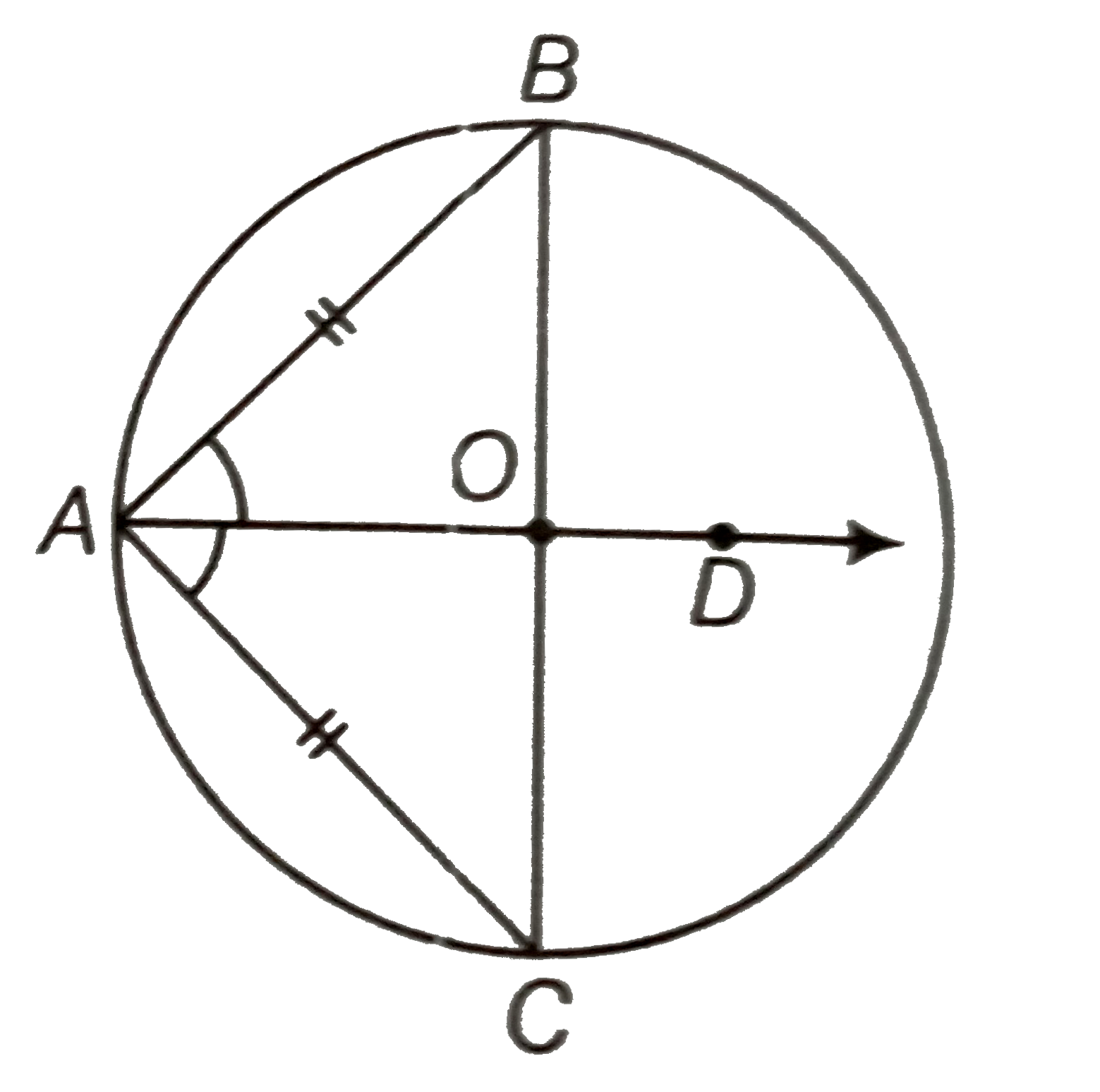Doubtnut App - Get 50 Lakh+ video solutions better than GoogleInstall Now

# Two chords AB and AC of a circle are equal. Prove that the centre of the circle lies on the angle bisector of ∠BAC.

Text Solution
Verified by Experts

## Given AB and AC are two equal chords whose centre is O. To prove Centre O lies on the bisector of ∠BAC. Construction Join BC, draw bisector AD of ∠BAC. Proof In ΔBAMandΔCAM, AB=AC [given] ∠BAM=∠CAM [given] and AM=AM [common side] ∴ΔBAM≅ΔCAM [by SAS congruence rule] ⇒BM=CM [by CPCT] and ∠BMA=∠CMA [by CPCT] So, BM=CMand∠BMA=∠CMA=90∘ So, AM is the perpendicular bisector of the chord BC. Hence, bisector of ∠BAC i.e., AM passes through the centre O.